×
Get Full Access to OleMiss - ECON 202 - Study Guide - Midterm
Get Full Access to OleMiss - ECON 202 - Study Guide - Midterm

×

OLEMISS / Engineering / ECON 202 / How do you calculate equivalent units of production?

How do you calculate equivalent units of production? Description

Description: These notes cover all of Chapters 16 - 19: Process Costing and Analysis, Activity Based Costing and Analysis, Cost Behavior and Cost-Volume-Profit Analysis, and Variable Costing and Analysis. I think this test is going to be pretty hard...Lots of formulas and calculations to remember...but I explain everything step-by-step, showing all calculations along the way. Hope it helps!
20 Pages 223 Views 6 Unlocks
Reviews

ACCY202

How do you calculate equivalent units of production?EXAM 2 Study Guide

Chapters 16 – 19

Prof. Barton

Chapter 16 – Process Costing and Analysis

Equivalent Units of Production (EUPs): a term for the number of units  that could have been started and completed given the cost incurred during the  period

• Note: When calculating EUPs and Cost per EUP, keep Direct Materials  separate from and Direct Labor and Overhead (Conversion) because % 0f completion may be different between them. If you want to learn more check out What type of tissue connects fibrous joints?

• Conversion Costs per Equivalent Unit of Production – combined costs of  direct labor and factory overhead per equivalent unit

• Why do we use EUPs? – they are used to assign costs to: 1. Good completed and transferred out to Finished Goods  Inventory (or another department)

Why do we need methods of allocated overhead?If you want to learn more check out What are the 6 soluble ionic compounds and their exceptions?

2. Ending Goods in Process Inventory for items that haven’t  finished production at the end of the period.

• Calculating EUPs with the weighted-average method:

EQUIVALENT UNITS OF PRODUCTION (EUPs) =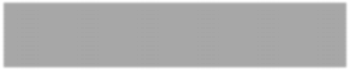# of UNITS COMPLETED AND TRANSFERRED OUT

+ # of UNITS IN ENDING WIP INVENTORY

• Process Cost Summary – reports manufacturing costs per equivalent unit of a  process or department

What is the predicted income from a predicted level of salesWe also discuss several other topics like What started the archaic period?

Computing EUPs in a problem:

Equivalent Units of Production Direct Materials  Conversion Units Completed and Transferred Out (# of units x  (# of units x 100%)  100%)

+ Units in Ending WIP

Direct Materials (# of units x

% completion)

Conversion (# of units x % completion)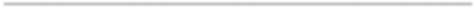Equivalent Units of Production = (ADD^) (and) (ADD^)

ACCY202

EXAM 2 Study Guide

Chapters 16 – 19

Prof. Barton

Computing Cost per EUP in a problem:

Cost Per Equivalent Unit of Production Direct Materials Conversion Costs of Beginning WIP inventory (Given) (Given) + Costs Incurred this period (Given – “Direct  (Given – “Direct  Materials Added”) Labor Added” +  If you want to learn more check out What are the disadvantages of public opinion?

Applied”)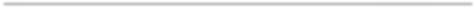Total Costs (^) (^) ÷ Equivalent Units of Production (Computed above!) (Computed  above!) If you want to learn more check out What is systole?Costs per Equivalent Unit of Production (Cost per EUP) (Cost per EUP)

Journal Entries to record Direct Materials and Direct Labor when they enter  production:

Work in Process Inventory – Dept. \$\$\$

(i.e. Raw Materials Inventory) \$\$\$

(OR) (i.e. Factory Payroll Payable) \$\$\$

Journal Entries to record Overhead Expenses like Indirect Materials, Indirect  Labor, and other OH expenses:

(i.e. Prepaid Insurance) \$\$\$

(i.e. Utilities Payable) \$\$\$

(i.e. Accumulated Depreciation) \$\$\$

Journal Entry to record Goods Completed and Transferred Out to Finished  Goods Inventory:

Finished Goods Inventory \$\$\$

Work in Process Inventory – Dept. \$\$\$

ACCY202

EXAM 2 Study Guide

Chapters 16 – 19

Prof. Barton

Chapter 16 Practice QUESTION!

Refer to the following information about the Assembly Department in Lombard Luxury Exports for the month of October: We also discuss several other topics like What is the meaning of supply?

Beginning goods in process inventory:

Physical units 10,000 units

% complete for materials 75%

% complete for labor and overhead 15%

Materials cost from September \$175,000

Labor and overhead cost from September \$41,500

Product started:

Physical units 40,000 units

Ending goods in process inventory:

Physical units 12,000 units

% complete for materials 50%

% complete for labor and overhead 10%

Manufacturing costs for October:

Materials \$375,000

ACCY202

EXAM 2 Study Guide

Chapters 16 – 19

Prof. Barton

1. Assume that the Assembly Department of Lombard Luxury Exports uses the weighted average method. What is the cost of all units that were completed and transferred to finished goods in October? What is the total cost of ending goods in process?

Cost of Units Completed

Transferred Out Physical Units Percent of Completion Equivalent Units Direct Materials:

Completed Units 7,500 units 100% 7,500 EUPs Ending Goods in Process 12,000 units 50% 6,000 EUPs Total Units 53,500 EUPs

Completed Units 1,500 units 100% 1,500 EUPs Ending Goods in Process 12,000 units 10% 1,200 EUPs Total Units 42,700 EUPs Direct Materials Cost:

Direct Materials in Beginning

Goods in Process \$175,000

During October \$375,000

Total Direct Material Costs \$550,000 Cost Per Equivalent Unit for Materials \$10.28 per EUP Direct Labor and Overhead Cost:

Beginning Goods in Process \$41,500

During October \$105,500

Total Direct Labor and Overhead Costs \$147,000 Cost Per Equivalent Unit for Direct Labor and

Assignment of Costs to

Finished Goods Equivalent Units Cost per EUP Total Cost Cost of goods completed and transferred

Direct materials 7,500 EUPs \$10.28 per EUP \$77,100 Direct labor and overhead 1,500 EUPs \$3.44 per EUP \$5,160 Total cost of goods completed \$82,260 Cost of goods in ending goods in process

Direct materials 6,000 EUPs \$10.28 per EUP \$61,680 Direct labor and overhead 1,200 EUPs \$3.44 per EUP \$4,128 Total costs of goods in process \$65,808

Journal Entry to transfer Completed

Units to Finished Goods Inventory:

Date Finished Goods Inventory \$148,068 Work in Process Inv. – Assembly Dept. \$148,068

ACCY202

EXAM 2 Study Guide

Chapters 16 – 19

Prof. Barton

Chapter 17 – Activity-Based Costing and Analysis

Why do we need methods of allocated overhead? – Because we need a way to  assign the right amount of overhead to the amount of production done in the  factory.

Plantwide Rate One Rate Volume-based measures  (direct labor hours,

machine hours)

Departmental Rate Two or more Rates Volume-based measures  (direct labor hours,

machine hours)

Activity-Based Costing At Least Two Rates Activities that drive costs  (batches, purchase orders)

• Plantwide Overhead Rate Method –

o The usefulness of this method depends on the assumption that  overhead costs change with the allocation base (such as  direct labor hours), and all products use overhead costs in the  same proportions.

o Not reasonable to use when a company has many different products,  or those with products that use overhead costs in very different  ways.

TOTAL BUDGETED OVERHEAD COSTS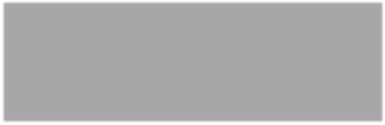÷ TOTAL BUDGETED (MACHINE or DIRECT LABOR) HOURS

x (MACHINE or DIRECT LABOR) HOURS PER UNIT

= OVERHEAD ALLOCATED TO EACH UNIT OF PRODUCT

ACCY202

EXAM 2 Study Guide

Chapters 16 – 19

Prof. Barton

• Departmental Overhead Rate Method -

o Assumes that different products are similar in volume,  complexity, and batch size, and that the departmental  overhead costs are directly proportional to the department  allocation base (such as direct labor hours or machine hours).

o Not reasonable when products differ in batch size and complexity,  because they would usually consume different amounts of overhead  costs. This method would fail to allocate overhead costs that are not  driven by production volume.

TOTAL BUDGETED DEPARTMENTAL OVERHEAD COST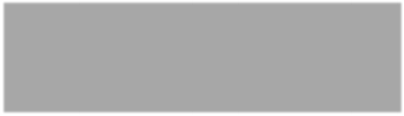÷ TOTAL AMOUNT OF DEPARTMENTAL ALLOCATION BASE

x (MACHINE or DIRECT LABOR) HOURS PER UNIT

= OVERHEAD ALLOCATED TO EACH UNIT OF PRODUCT

• Activity-Based Costing (ABC) Rate Method -

o Assigns overhead costs based on individual activities (tasks,  operations, or procedures where costs are incurred) instead of a  single rate, making it often more accurate than departmental or  plantwide rates, easier to control costs, and allows for better  management and to focus on relevant factors.

o When two activities have the same cost-driver (batches, purchase  orders, square feet), you can group them together in the same  pool.

OVERHEAD COSTS ASSIGNED TO POOL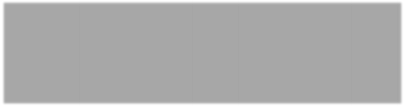÷ EXPECTED ACTIVITY LEVEL

= COST POOL ACTIVITY RATE

ACTIVITIES CONSUMED x ACTIVITY RATE

= OVERHEAD FROM POOL ALLOCATED TO SPECIFIC PRODUCT

ACCY202

EXAM 2 Study Guide

Chapters 16 – 19

Prof. Barton

Chapter 17 Practice question!

A sign company produces 3 products: Hotty Toddy, Roll Tide, and Geaux Tigers. Historically,  the company has used a single, plantwide overhead rate based upon direct labor hours to  allocate overhead costs to inventory. There are two departments in the company: Print and  Finish. The company has experienced growth and is interested in implementing more  sophisticated inventory cost techniques and identifying ways to improve their profit margins.

The company is considering the departmental overhead rate method of applying overhead costs. They budget that the Print Department will incur about \$50,000 overhead costs and will use machine hours to allocate overhead costs. They budget that the Finish Department

will incur about \$40,000 overhead costs and will use direct labor hours to allocate overhead costs. Estimated machine hours and direct labor hour usage is below:

Hotty Toddy Roll Tide Geaux Tigers

Machine hours 2,500 1,650 2,100  Direct labor hours 1,750 1,050 1,200

What amount of overhead would be applied to Hotty Toddy, Roll Tide, and Geaux Tigers  inventory in the coming period using departmental rates to apply overhead?

Hotty Toddy Roll Tide Geaux Tigers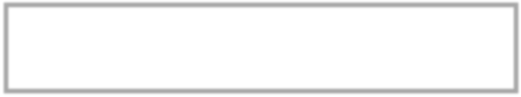Print Department – \$20 per MH \$30.30 per MH \$23.81 per MH (\$50,000 ÷ 2,500 MH) (\$50,000 ÷ 1,650 MH) (\$50,000 ÷ 2,100 MH)

Finish Department – \$22.86 per DLH \$38.10 per DLH \$33.33 per DLH (\$40,000 ÷ 1,750 DLH) (\$40,000 ÷ 1,050 DLH) (\$40,000 ÷ 1,200 DLH)

Total \$42.86 appl. OH \$68.40 appl. OH \$57.14 appl. OH

The Director of Operations has identified 4 production activities and their cost drivers and has computed the cost per cost driver for each. The budgeted rates and usage levels for each  are below:

Budgeted Activity Drivers

Activity Cost/Driver Hotty Toddy Roll Tide Geaux Tigers Canvas cutting (MH) \$15.00 800 650 715 Screen pressing (DLH) \$17.50 550 285 475 Stitching (MH) \$15.00 420 300 350 Packaging (DLH) \$17.50 495 215 350

Assume that the company chooses activity-based costing to assign overhead costs to inventory. What are the cost pools and what amounts of budgeted overhead would be allocated to Hotty Toddy, Roll Tide, and Geaux Tigers inventory?

ACCY202

EXAM 2 Study Guide

Chapters 16 – 19

Prof. Barton

Activity Cost Pool Cost/Driver Hotty Toddy Roll Tide Geaux Tigers Canvas Cutting \$15 per MH \$12,000 \$9,750 \$10,725 Stitching \$6,300 \$4,500 \$5,250 Screen Pressing \$17.50 per DLH \$9,625 \$4,987.50 \$8,312.50 Packaging \$8,662.50 \$3,762.50 \$6,125 Totals \$36,587.50 \$23,000 \$30,412.50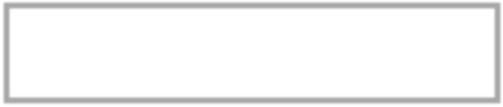ACCY202

EXAM 2 Study Guide

Chapters 16 – 19

Prof. Barton

CHAPTER 18 – COST BEHAVIOR AND COST-VOLUME-PROFIT ANALYSIS

Cost-Volume-Profit (CVP) Analysis - requires management to classify  costs as either fixed or variable with respect to production or sales volume,  within the relevant range of operations.

• Relevant range of operations – normal operating range for a  business, which excludes projected times of unusually high and low  business

• The Five Cost-Volume-Profit Analysis Assumptions:  o The behavior of both costs and revenues is linear throughout the  relevant range of the activity index.

o Costs can be classified accurately as either variable or fixed. o All units produced are sold.

o Changes in activity are the only factors that affect costs. o When more than one type of product is sold, the percentage of  each product in total sales will remain constant.

▪ Note: the CVP Analysis may be inaccurate if all five

assumptions are not valid.

• CVP Graphs (break-even graphs)– shows the relationship between costs,  volume, and profits

o Horizontal Axis – number of units produced and sold o Vertical Axis – dollars of sales and costs

▪ Fixed costs are shown with a horizontal line that runs  through the y-axis at the point where the sum of fixed costs

lies.

▪ Variable plus fixed costs are represented on the graph  with a diagonal line (positive slope) starting from the  fixed cost point because total costs equal fixed costs at zero  volume. The slope of this total cost line equals the

variable cost per unit.

▪ The sales line starts at zero because there are zero units  sold and zero dollars of sales at the beginning of the period.

The slope of this line is equal to the selling price per  unit.

o The lines of the graph depict both sales and costs at different output  levels

ACCY202

EXAM 2 Study Guide

Chapters 16 – 19

Prof. Barton

• Types of costs in a CVP Analysis:

o Fixed Costs – remain unchanged despite variation in the volume of  activity within a relevant range

▪ Common fixed costs: rent, depreciation, property taxes, office  salaries, and many service department costs

▪ Usually represented as a horizontal line, when production  volume and production costs are graphed.

o Variable Costs – change in proportion to changes in volume of  activity

▪ Common variable costs: direct materials, direct labor (if  employees are paid per unit), sales commissions, shipping  costs, and some overhead costs

▪ Usually represented by a straight diagonal line (constantly  increasing, positive slope) starting from zero, when production  volume and costs are graphed.

o Step-wise (stair-step) Costs – reflects a step pattern in costs ▪ Common step-wise costs: salaries of production supervisors (if  production volume increases to where another supervisor

must be hired)

▪ When graphed on a production volume-cost graph, it will look  like steps, starting from zero. The flat tops of the steps will  signify the different relevant ranges.

o Curvilinear Costs – reflects a non-constant linear increase or  decrease in costs

▪ Common curvilinear costs: total direct labor costs (when  employees are paid by the hour)

▪ On a production volume-cost graph, the nonlinear graph will  have a flatter slope when output is higher and will have a  steeper slope when there are inefficiencies (such as too  many employees getting in each other’s way).

▪ Often treated as variable costs within a relevant range, which  is reasonable for most types of curvilinear costs.

• Methods for Determining Cost Behavior:

o Scatter Diagrams –

▪ Display past cost and unit data in graphical form

▪ Units are plotted on the horizontal axis and costs are plotted  on the vertical axis

ACCY202

EXAM 2 Study Guide

Chapters 16 – 19

Prof. Barton

▪ Each individual point reflects the cost and number of units for  a prior period

▪ Estimated Line of Cost Behavior – straight line that best  visually “fits” the scattered points and reflecting the

relationship between cost and unit volume

• How to determine the slope of the estimated line of cost  behavior –

SLOPE =(FINAL COSTS – INITIAL COSTS)

÷ (FINAL UNITS – INITIAL UNITS)

o High-Low Method –

▪ Estimates the cost equation by using two points on a graph,  the highest volume level and the lowest volume level; note:  this doesn’t reflect the level of costs, it represents production  volume

▪ The slope of the line connecting the two points is the variable  cost per unit

• This method uses the same formula as the scatter

diagrams to find the slope of the line on the graph.

▪ To estimate fixed cost per unit, we say that…

TOTAL COSTS =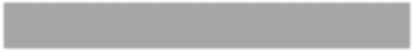FIXED COST + (VARIABLE COST PER UNIT x # OF UNITS)

o Least-Squares Regression –

▪ A statistical method for identifying cost behavior of total fixed  costs and variable unit cost

▪ Don’t need to know how to work these…just know what it is. o Comparison of Cost Estimation Methods:

o Estimates from a scatter diagram are based on visual fit,  and therefore is subject to interpretation.

o Estimates from the high-low method use only two sets of  values corresponding to the lowest and highest unit volumes;  however, sometimes these two extreme activity levels do not  reflect the more usual conditions that are likely to recur.

ACCY202

EXAM 2 Study Guide

Chapters 16 – 19

Prof. Barton

This method is easy to apply, and useful for obtaining a quick cost equation estimate.

o Estimates from the least-squares regression method use a  statistical and all available data points.

o All methods use past data, thus cost estimates resulting from  these methods are only as good as the data used for

estimation.

• Contribution Margin and Break-Even Analysis:

o Contribution Margin – the amount by which a product’s unit  selling price exceeds its total variable cost per unit

CONTRIBUTION MARGIN PER UNIT =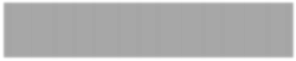SELLING PRICE PER UNIT

– TOAL VARIABLE COST PER UNIT

o Contribution Margin Ratio – the percent of a unit’s selling  price that exceeds total unit variable cost; the percent of each  sales dollar that remains after deducting the total unit variable  cost

CONTRIBUTION MARGIN RATIO =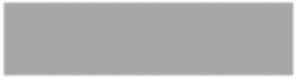CONTRIBUTION MARGIN PER UNIT

÷ SELLING PRICE PER UNIT

o Breakeven point – the sales level at which a company neither  earns a profit not incurs a loss; when total sales cover total costs

BREAK EVEN POINT (in units) =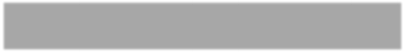FIXED COSTS ÷ CONTRIBUTION MARGIN PER UNIT

BREAK EVEN POINT (in dollars) =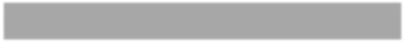FIXED COSTS ÷ CONTRIBUTION MARGIN RATIO

ACCY202

EXAM 2 Study Guide

Chapters 16 – 19

Prof. Barton

o Margin of Safety – the excess of expected sales over the break even sales level

MARGIN OF SAFETY (%) =

(EXPECTED SALES – BREAK EVEN SALES)

÷ EXPECTED SALES

o Contribution Margin Income Statement for break-even sales –

COMPANY

Contribution Margin Income Statement (at Break-Even)

For Month Ended January 1, 2016

Sales (# of units x selling price per unit) - Variable Costs (# of units x variable cost per unit)

Contribution Margin (sales – variable costs)

- Fixed Costs (given)

Net Income (contribution margin – fixed costs)

• Target Pre-tax Income –

o Often, managers will ask, “What is the predicted income from a  predicted level of sales?” To determine this, we look at the CVP  Analysis, and the relation of sales, variable costs, contribution  margin, and fixed costs.

o Computing Income from Sales and Costs:

SALES – VARIABLE COSTS = CONTRIBUTION MARGIN CONTRIBUTION MARGIN – FIXED COSTS = INCOME (Pre-Tax)

ACCY202

EXAM 2 Study Guide

Chapters 16 – 19

Prof. Barton

o Computing Sales for a Target Income:

▪ Many companies’ annual plans are based on certain income  targets (sometimes called budgets)

DOLLAR SALES AT TARGET INCOME =

(FIXED COSTS + TARGET INCOME)

÷ CONTRIBUTION MARGIN RATIO

UNIT SALES AT TARGET INCOME =

(FIXED COSTS + TARGET INCOME)

÷ CONTRIBUTION MARGIN PER UNIT

ACCY202

EXAM 2 Study Guide

Chapters 16 – 19

Prof. Barton

Chapter 18 Practice Question!

Best Co. sells two products, vests and jackets. For every vest sold, three jackets are sold (1:3 sales ratio). Best Co.'s total fixed costs are \$1,056,250. Selling prices and cost information for  both products follow:

Product Unit Sales Price Variable Costs per Unit Units Sold in 2015 Vests \$75 20 18,000 Jackets \$150 60 54,000

a.) Calculate break-even units of both vests and jackets.

Vests:

Selling Price – Total Variable Costs Per Unit = Contribution Margin Per Unit \$75 – 20 = \$55

Fixed Cost ÷ Contribution Margin Per Unit = Break-even Point in Units \$1,056,250 ÷ \$55 = 19,205 vests

Jackets:

Selling Price – Total Variable Costs Per Unit = Contribution Margin

\$150 – 60 = \$90

Fixed Cost ÷ Contribution Margin Per Unit = Break-even Point in Units \$1,056,250 ÷ \$90 = 11,737 jackets

b.) Calculate Best Co.’s pretax income for 2015.

Total Sales – Total Variable Costs = Contribution Margin

((\$75 x 18,000) + (\$150 x 54,000)) – ((20 x 18,000) + (60 x 54,000)) =  (\$1,350,000 + \$8,100,000) – (360,000 + 3,240,000) =

\$9,450,000 – 3,600,000 = \$5,850,000

Contribution Margin – Fixed Costs = Pre-Tax Income

\$5,850,000 - \$1,056,250 = \$4,793,750

c.) Calculate the 2015 margin of safety for jackets (rounded to the nearest whole percentage).

Jackets:

Contribution Margin Per Unit ÷ Selling Price Per Unit = Contribution Margin Ratio \$90 ÷ \$150 = 60%

Fixed Costs ÷ Contribution Margin Ratio = Break-even Point in Dollars \$1,056,250 ÷ 60% = \$1,775,416.67

(Expected Sales – Break-Even in Dollars) ÷ Expected Sales = Margin of Safety (%) (\$8,100,000 - \$1,775,416.67) ÷ \$8,100,000 = 78%

ACCY202

EXAM 2 Study Guide

Chapters 16 – 19

Prof. Barton

CHAPTER 19 – Variable Costing and Analysis

Absorption Costing (full costing) –

• All manufacturing costs are assigned to products, consisting of direct  materials, direct labor, and factory overhead, both variable and fixed.

DIRECT MATERIALS + DIRECT LABOR

= INVENTORY under ABSORBTION COSTING

• “Traditional Method”; widely used for GAAP (external and  tax reporting), but can result in misleading product cost

• Fixed overhead is dispensed at the time the units are sold (as a  component of cost of goods sold), which means fixed overhead is  deferred to a future period.

• Absorption Costing Income Statement:

COMPANY

Income Statement (Absorption Costing)

For Year Ended January 1, 2016

Sales (# of units sold x selling price) - Cost of Goods Sold (# of units sold x (direct material cost  per unit + direct labor costs per unit +

variable overhead costs per unit +

(fixed overhead cost ÷ # of units

produced in period))

Gross Margin (sales – cost of goods sold) - Selling and Administrative Expenses (fixed S&A expenses + (# of units  produced x S&A variable costs per

unit))

Net income (gross margin – selling and  administrative expenses)

ACCY202

EXAM 2 Study Guide

Chapters 16 – 19

Prof. Barton

Variable Costing – only costs that change in total with changes in production  level are included in product costs

DIRECT MATERALS + DIRECT LABOR + VARIABLE OVERHEAD = INVENTORY under VARIABLE COSTING

• Fixed overhead is excluded from the from product costs under  variable costing because it will not change with changes in production • Treats fixed factory overhead as a period cost, where it is expensed in  the period it is incurred.

• Variable Costing Income Statement:

COMPANY

Income Statement (Variable Costing)

For Year Ended January 1, 2016

Sales (# of units sold x selling price) - Variable Expenses

Variable Production Costs (# of units sold x (direct material cost  per unit + direct labor cost per unit +

variable over head costs per unit))

Variable Selling and

Administrative Expenses (# of units sold x variable S&A costs  per unit)

Contribution Margin (sales – (variable production  costs + variable S&A Expenses))

- Fixed Expenses

Administration Expenses (fixed s&a expenses, given)

Net Income (contribution margin – (fixed overhead  + fixed S&A expenses))

ACCY202

EXAM 2 Study Guide

Chapters 16 – 19

Prof. Barton

• Variable Costing and Special Orders:

o In the case of excess production capacity, increases in production  levels would increase variable production costs, but not fixed  production costs. A manager would then need to accept special  orders provided that the special order price exceeds the  variable cost.

Converting Variable Costing Net Income to Absorption Costing  Net Income –

• Under absorption costing, income is higher when more units are  produced relative to units sold and is lower when fewer units  are produced than sold.

INCOME UNDER VARIABLE COSTING

+ FIXED OVERHEAD COST IN ENDING INVENTORY

– FIXED OVERHEAD COST IN BEGINNING INVENTORY = INCOME UNDER ABSORPTION COSTING

ACCY202

EXAM 2 Study Guide

Chapters 16 – 19

Prof. Barton

Chapter 19 Practice Question!

Elease, Inc. reports the following product cost information:

Units Produced 80,000 units

Units Sold 65,000 units

Unit Sales Price \$98 per unit

Direct Labor \$16.50 per unit

Direct Materials \$28 per unit

Variable Selling and

Fixed Selling and

a.) Prepare the absorption costing income statement.

Elease, Inc.

Income Statement (Absorption)

For Year Ended …..

Sales \$6,370,000

(65,000 x \$98)

- Cost of Goods Sold \$4,257,500

(65,000 x (28 + 16.50 + (640,000 ÷ 80,000)

+ (1,040,000 ÷ 80,000))

Gross Margin \$2,112,500

(6,370,000 – 4,257,500)

- Selling and Administration Expenses \$740,000

(465,000 + 275,000)

Net Income \$1,372,500

(2,112,500 – 740,000)

ACCY202

EXAM 2 Study Guide

Chapters 16 – 19

Prof. Barton

b.) Prepare the variable costing income statement.

Elease, Inc.

Income Statement (Variable)

For Year Ended ….

Sales \$6,370,000

(65,000 x 98)

- Variable Production Costs \$3,412,500

(65,000 x (28 + 16.50 + 8))

- Variable Selling and

Contribution Margin \$2,682,500

(6,370,000 – 3,412,500 – 275,000)

- Fixed Selling and

Net Income \$1,177,500

(2,682,500 – 1,040,000 – 465,000)

c.) Determine the cost of ending inventory (in total) using absorption costing.

80,000 x 28 = 2,240,000

80,000 x 16.50 = 1,320,000

640,000

+ 1,040,000

\$5,240,000 = ending inventory under absorption costing

d.) Determine the cost of ending inventory (in total) using variable costing.

80,000 x 28 = 2,240,000

80,000 x 16.50 = 1,320,000

+ 640,000

\$4,200,000 = ending inventory under variable costing

Page ExpiredIt looks like your free minutes have expired! Lucky for you we have all the content you need, just sign up here